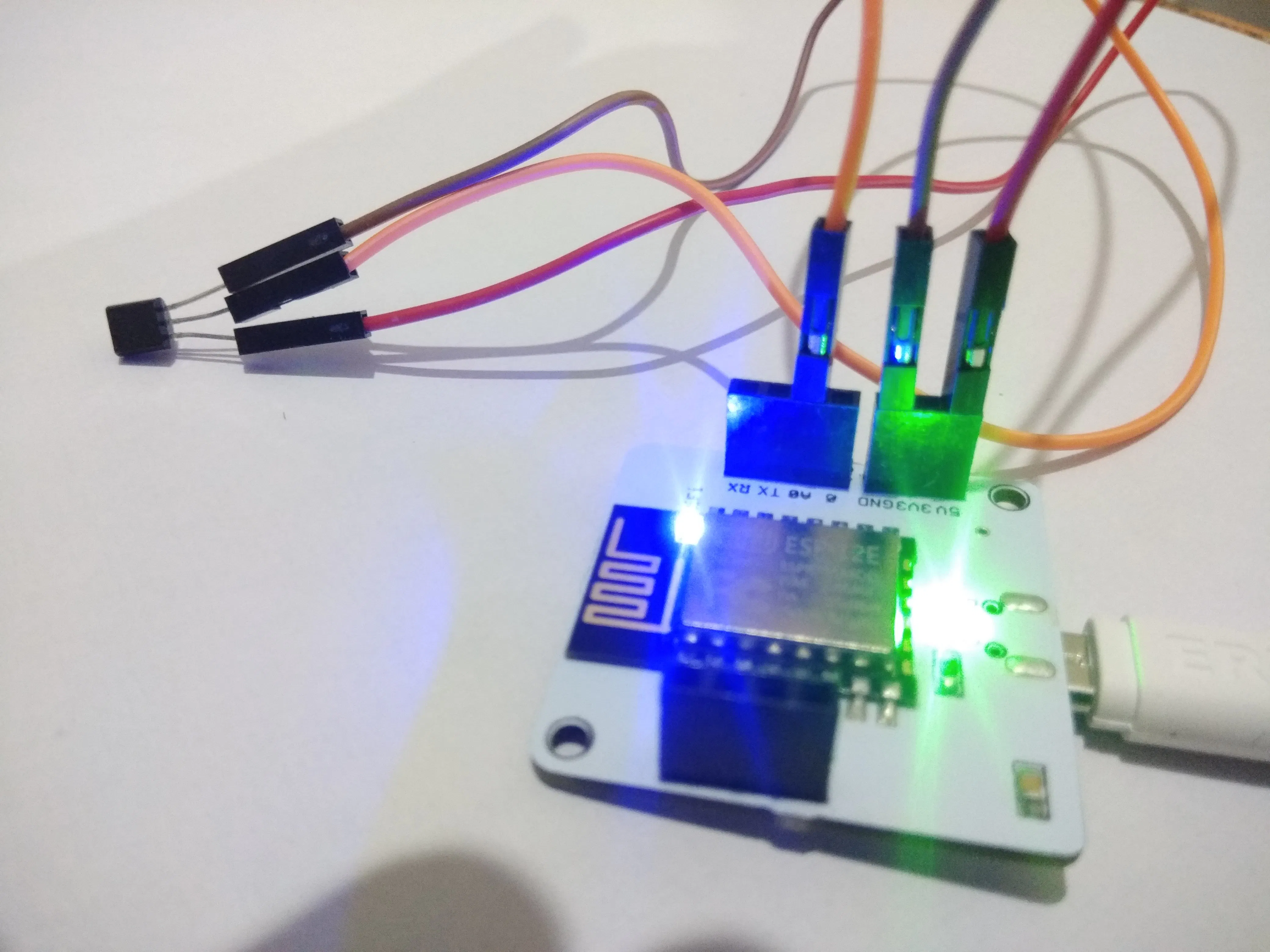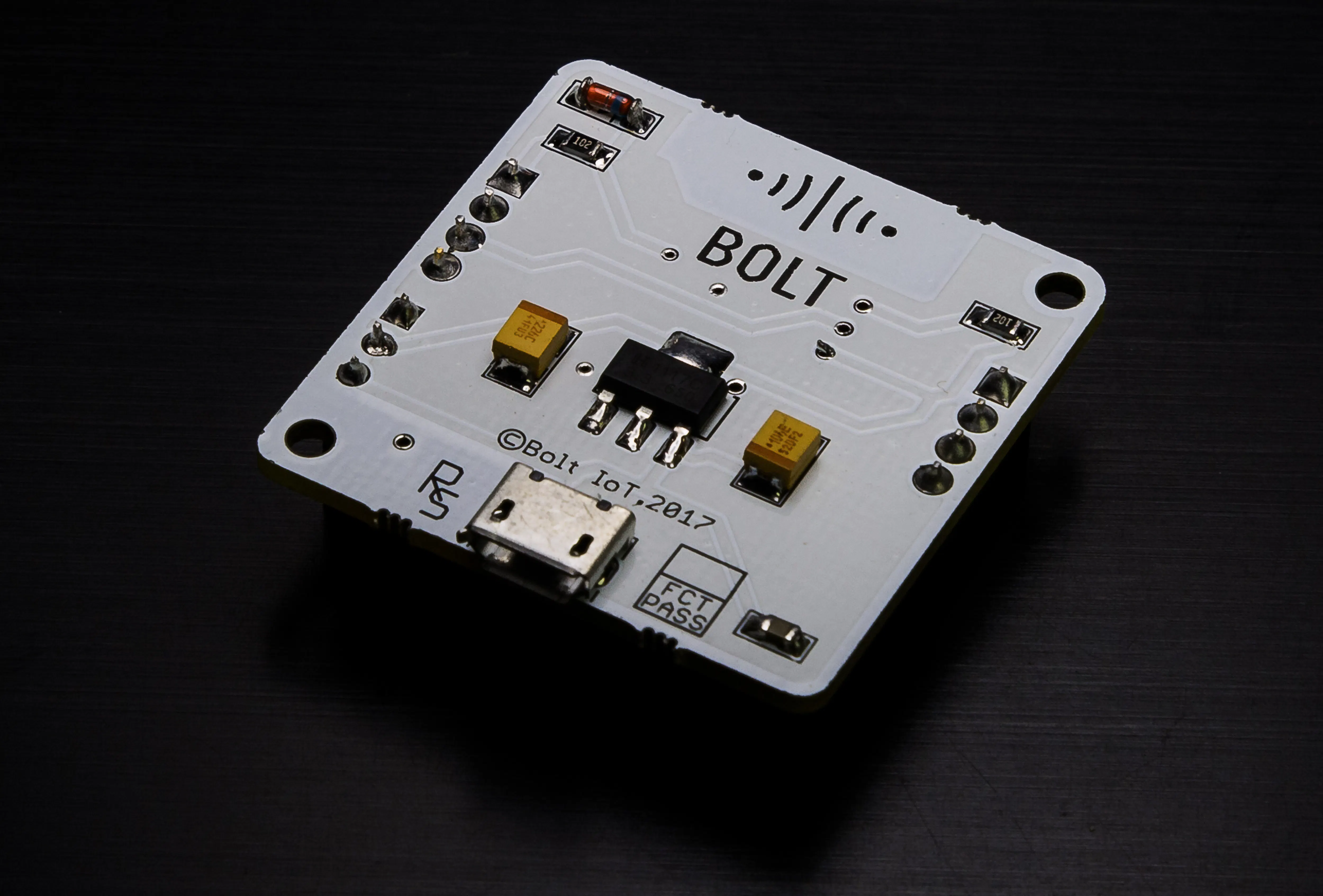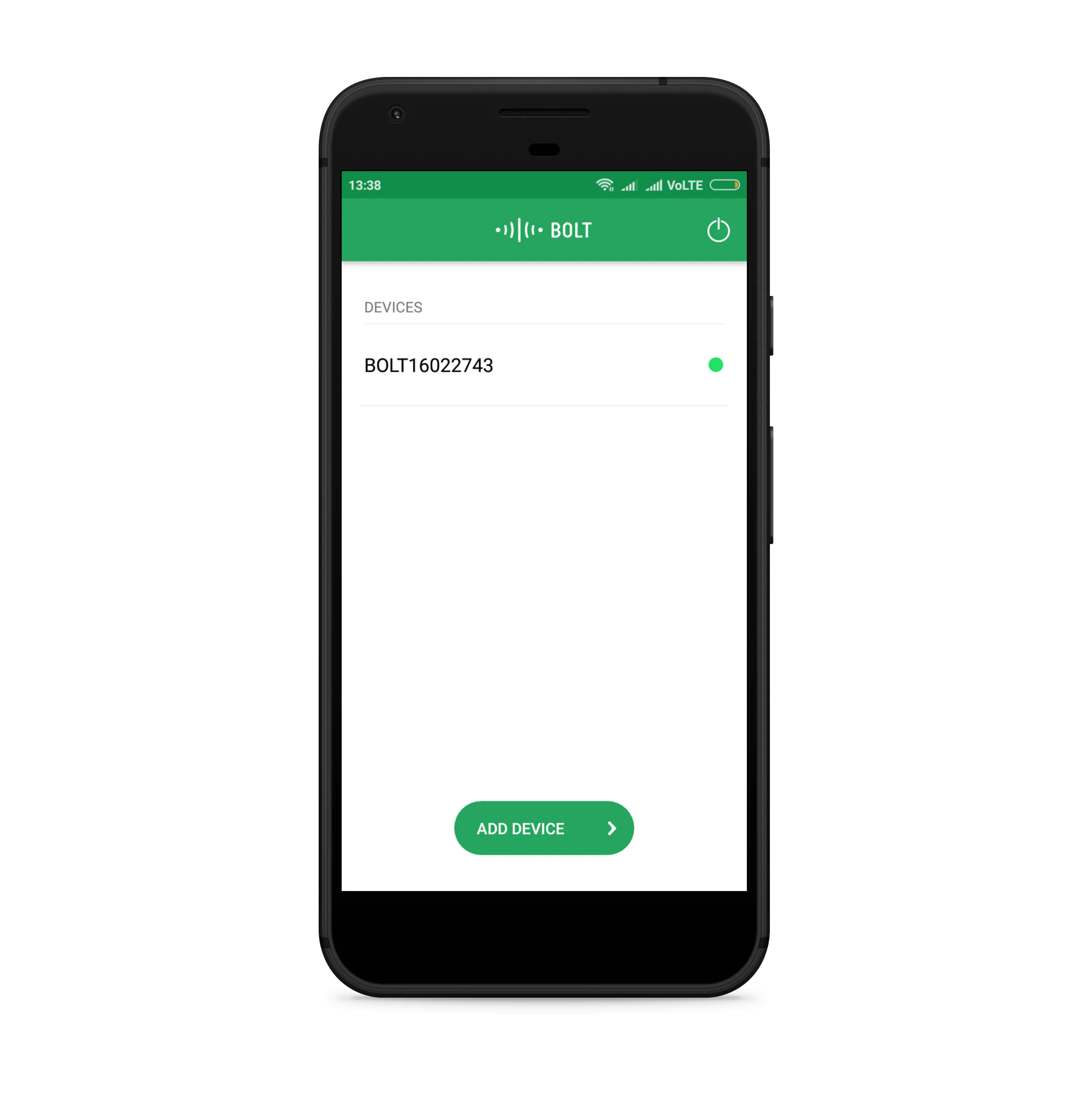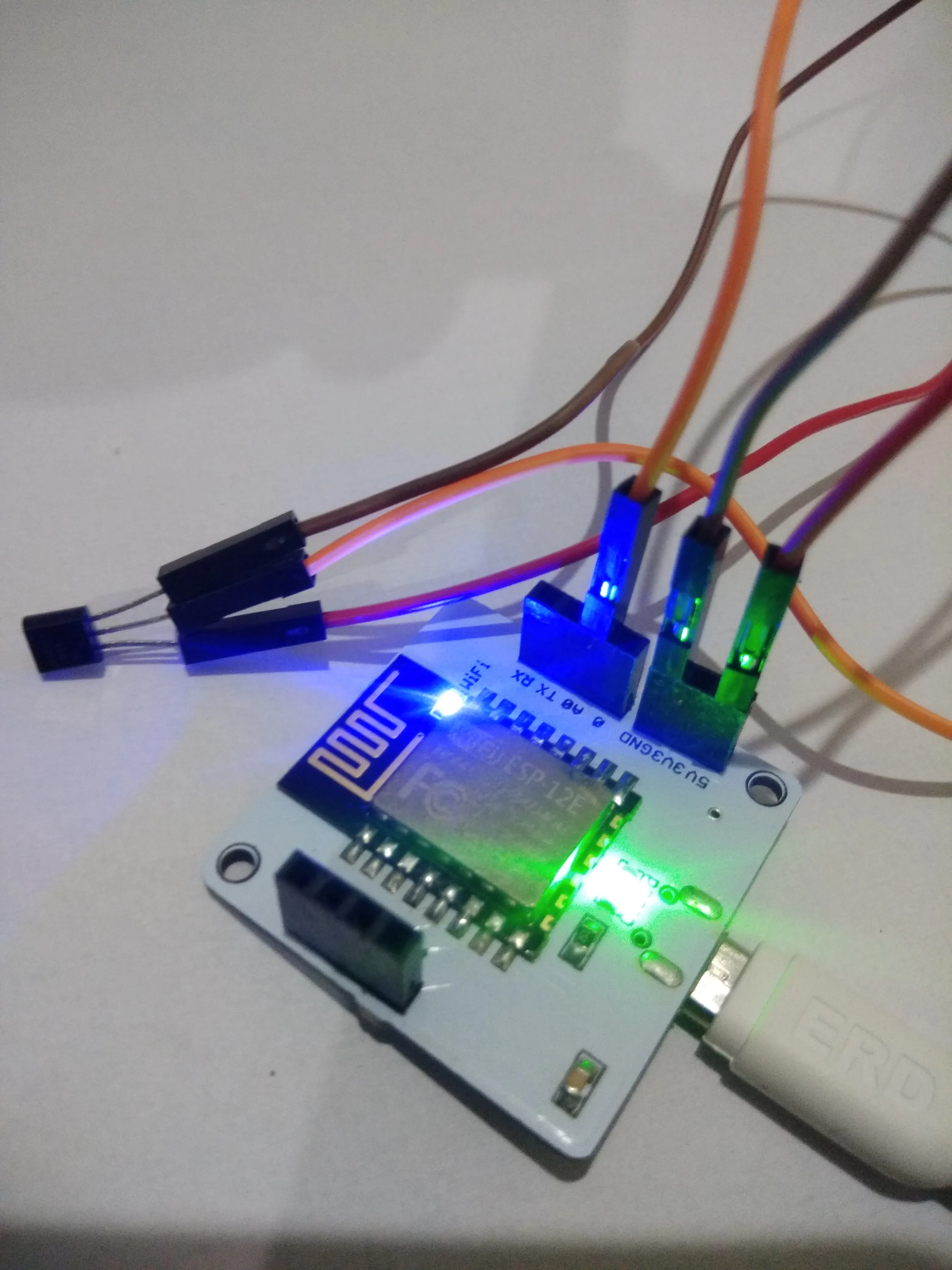Published

# Capstone Project

Analyze temperature change and send an email if it crosses the threshold. It also does anomaly detection.

IntermediateFull instructions provided5 hours499## Things used in this project

### Hardware componentsBolt IoT Bolt WiFi Module
×1Temperature Sensor
×1Male/Female Jumper Wires
×1

### Software apps and online servicesBolt IoT Bolt CloudBolt IoT Android App

## Schematics

### Circuit## Code

### email_conf

Python
```gmail_user = 'Sender Email id'
API_KEY = 'API Key'
DEVICE_ID = 'Device ID'
FRAME_SIZE = 5
MUL_FACTOR = 6
```

### email_send

Python
```import smtplib
import email_conf,json,time
from boltiot import Bolt
import math, statistics

minimum_limit = 50 #the minimum threshold of light value
maximum_limit = 100 #the maximum threshold of light value

mybolt = Bolt(email_conf.API_KEY, email_conf.DEVICE_ID)

def compute_bounds(history_data,frame_size,factor):
if len(history_data)<frame_size :
return None

if len(history_data)>frame_size :
del history_data[0:len(history_data)-frame_size]
Mn=statistics.mean(history_data)
Variance=0
for data in history_data :
Variance += math.pow((data-Mn),2)
Zn = factor * math.sqrt(Variance / frame_size)
High_bound = history_data[frame_size-1]+Zn
Low_bound = history_data[frame_size-1]-Zn
return [High_bound,Low_bound]

history_data=[]

while True:
print ("Sensor value is: " + str(data['value']))
try:
sensor_value = int(data['value'])

#Anomaly Detection
bound = compute_bounds(history_data,email_conf.FRAME_SIZE,email_conf.MUL_FACTOR)
if not bound:
required_data_count=email_conf.FRAME_SIZE-len(history_data)
print("Not enough data to compute Z-score. Need ",required_data_count," more data points")
history_data.append(int(data['value']))
time.sleep(10)
continue

try:
if sensor_value > bound :
print("bound = ",bound)
print ("Someone has opened the fridge door")
print("This is the response ",response)
elif sensor_value < bound:
print("bound = ",bound)
print ("Someone has opened the fridge door")
print("This is the response ",response)
history_data.append(sensor_value);

except Exception as e:
print ("Error",e)
continue

#Email send if temprature crossed threshold
if sensor_value > maximum_limit or sensor_value < minimum_limit:
print("Tempurature Crosses Threshold")
smtpserver=smtplib.SMTP("smtp.gmail.com",587)
smtpserver.ehlo()
smtpserver.starttls()
smtpserver.ehlo()
smtpserver.sendmail(email_conf.gmail_user,email_conf.to,msg)
print('Email Send Successfully! :)')
smtpserver.close()
except Exception as e:
print ("Error occured: Below are the details")
print (e)
time.sleep(10)
```

## Credits

### Omkar Kolate

1 project • 0 followers
I am a final year Computer Engineering student.
Thanks to Bolt IoT.